Courses

# RD Sharma Solutions -Ex-20.5, Mensuration, Class 6, Maths Class 6 Notes | EduRev

## RD Sharma Solutions for Class 6 Mathematics

Created by: Abhishek Kapoor

## Class 6 : RD Sharma Solutions -Ex-20.5, Mensuration, Class 6, Maths Class 6 Notes | EduRev

The document RD Sharma Solutions -Ex-20.5, Mensuration, Class 6, Maths Class 6 Notes | EduRev is a part of the Class 6 Course RD Sharma Solutions for Class 6 Mathematics.
All you need of Class 6 at this link: Class 6

Mark the correct alternative in each of the following:

Q. 1. The sides of a rectangle are in the ratio 5 : 4. If its perimeter is 72 cm, then its length is

(a) 40 cm (b) 20 cm (c) 30 cm (d) 60 cm

Explanation:

Let the sides of the rectangle be 5x and 4x. (Since, they are in the ratio 5 : 4)

Now, perimeter of rectangle = 2 (Length + Breadth)

72 = 2 (5x + 4x)

72 = 2 × 9x

72 = 18x

x = 4

Thus, the length of the rectangle = 5x = 5 × 4 = 20 cm

Q. 2. The cost of fencing a rectangular field 34 m long and 18 m wide at As 2.25 per metre is

(a) Rs 243 (b) Rs 234 (c) Rs 240 (d) Rs 334

Explanation:

For fencing the rectangular field, we need to find the perimeter of the rectangle.

Length of the rectangle = 34 m

Breadth of the rectangle = 18 m

Perimeter of the rectangle = 2 (Length + Breadth) = 2 (34 + 18) m = 2 × 52 m = 104 m

Cost of fencing the field at the rate of Rs. 2.25 per meter = Rs. 104 × 2.25 = Rs. 234

Q. 3. If the cost of fencing a rectangular field at Rs. 7.50 per metre is Rs. 600, and the length of the field is 24 m, then the breadth of the field is

(a) 8 m (b) 18 m (c) 24 m (d) 16 m

Explanation:

Cost of fencing the rectangular field = Rs. 600

Rate of fencing the field = Rs. 7.50 per m

Therefore, perimeter of the field = Cost of fencing / Rate of fencing = 600 / 7.50 = 80 m

Now, length of the field = 24 m

Therefore, breadth of the field = Perimeter / 2 - Length = 80 / 2- 24 = 16 m

Q. 4. The cost of putting a fence around a square field at As 2.50 per metre is As 200. The length of each side of the field is

(a) 80 m (b) 40 m (c) 20 m (d) None of these

Explanation:

Cost of fencing the square field = Rs. 200

Rate of fencing the field = Rs. 2.50

Now, perimeter of the square field = Cost of fencing / Rate of fencing = 200 / 2.50 = 80 m

Perimeter of square = 4 x Side of the square

Therefore, side of the square = Perimeter / 4 = 80 / 4 = 20 m

Q. 5. The length of a rectangle is three times of its width. If the length of the diagonal! is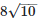, then the perimeter of the rectangle is

(a)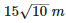(b)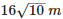(c)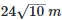(d) 64 m

Explanation:

Let us consider a rectangle ABCD.

Also, let us assume that the width of the rectangle, i.e., BC be x m.

It is given that the length is three times width of the rectangle.

Therefore, length of the rectangle, i.e., AB = 3x m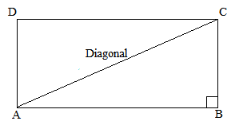Now, AC is the diagonal of rectangle.

In right angled triangle ABC.

AC2 = AB2 + BC2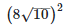= (3x)2 + x2

640 = 9x2 + x2

640 = 10x2

x2 = 640 / 10 = 64

x = 64 = 8 m

Thus, breadth of the rectangle = x = 8 m

Similarly, length of the rectangle = 3x = 3 x 8 = 24 m

Perimeter of the rectangle = 2 (Length + Breadth)

= 2 (24 + 8)

= 2 x 32 = 64 m

Q. 6. If a diagonal of a rectangle is thrice its smaller side, then its length and breadth are in the ratio

(a) 3 : 1 (b)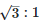(c)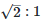(d)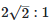Explanation:

Let us assume that the length of the smaller side of the rectangle, i.e., BC be x and length of the larger side , i.e., AB be y.

It is given that the length of the diagonal is three times that of the smaller side.

Therefore, diagonal = 3x = AC

Now, applying Pythagoras theorem, we get:

(Diagonal)2 = (Smaller side)2 + (Larger side)2(AC)2 = (AB)2 + (BC)2

(3x)2 = (x)2 + (y)2

9x2 = x2 + y2

8x2 = y2

Now, taking square roots of both sides, we get:

22 x = y

or, y / x = 22 / 1

Thus, the ratio of the larger side to the smaller side = 22 : 1

Q. 7. The ratio of the areas of two squares, one having its diagonal double than the other, is

(a) 1 : 2 (b) 2:3 (c) 3 : 1 (d) 4 : 1

Explanation:

Let the two squares be ABCD and PQRS. Further, the diagonal of square PQRS is twice the diagonal of square ABCD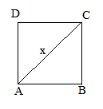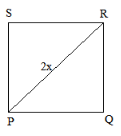PR = 2 AC

Now, area of the square =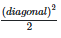Area of PQRS =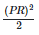Similarly, area of ABCD =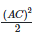According to the question:

If AC = x units, then, PR = 2x units

Therefore, Area of PQRS / Area of ABCD =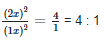Thus, the ratio of the areas of squares PQRS and ABCD = 4 : 1

Q. 8. If the ratio of areas of two squares is 225 : 256, then the ratio of their perimeters is

(a) 225 : 256 (b) 256 : 225 (c) 15:16 (d) 16 : 15

Explanation:

Let the two squares be ABCD and PQRS.

Further, let the lengths of each side of ABCD and PQRS be x and y, respectively.

Therefore Area of sq. ABCD / Area of sq. PQRS = x2 / y2

=> x2 / y2= 225 / 256

Taking square roots on both sides, we get:

x / y = 15 / 16

Now, the ratio of their perimeters:

Perimeter of sq. ABCD / Perimeter of sq. PQRS

= 4 × side of sq. ABCD / 4 × Side of sq. PQRS = 4x / 4y

Perimeter of sq. ABCD / Perimeter of sq. PQRS = x y

Perimeter of sq. ABCD / Perimeter of sq. PQRS = 15 / 16

Thus, the ratio of their perimeters = 15 : 16

Q. 9. If the sides of a square are halved, then its area

(a) remains same (b) becomes half (c) becomes one fourth

(d) becomes double

Explanation:

Let the side of the square be x.

Then, area = (Side x Side) = (x × x) = x2

If the sides are halved, new side = x / 2

Now, new area =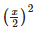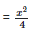It is clearly visible that the area has become one-fourth of its previous value.

Q. 10. A rectangular carpet has area 120 m2 and perimeter 46 metres. The length of its diagonal is

(a) 15 m (b) 16 m (c) 17 m (d) 20 m

Explanation:

Area of the rectangle = 120 m2

Perimeter = 46 m

Let the sides of the rectangle be l and b.

Therefore

Area = lb = 120 m2 ...(1)

Perimeter = 2 (l + b) = 46

Or, (l + b) = 46 / 2 =23 m ...(2)

Now, length of the diagonal of the rectangle = l2 + b2

So, we first find the value of (l2 + b2)

Using identity:

(l2 + b2) = (l + b)2 - 2 (lb) [From (1) and (2)]

Therefore

(l2 + b2) = (23)2 - 2 (120)

= 529 - 240 = 289

Thus, length of the diagonal of the rectangle = l2 + b2 = 289 = 17 m

Q. 11. If the ratio between the length and the perimeter of a rectangular plot is 1 : 3, then the ratio between the length and breadth of the plot is

(a) 1 : 2 (b) 2 : 1 (c) 3 : 2 (d) 2 : 3

Explanation:

It is given that Length of rectangle / Perimeter of rectangle = 1 / 3

=> l / (2l + 2b) = 1 / 3

After cross multiplying, we get:

3l = 2l + 2b

=> l = 2b

=> l / b= 2 / 1

Thus, the ratio of the length and the breadth is 2 : 1.

Q. 12. If the length of the diagonal of a square is 20 cm, then its perimeter is

(a)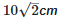(b) 40 cm (c)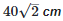(d) 200 cm

Answer: (c)Explanation:

Length of diagonal = 20 cm

Length of side of a square =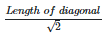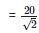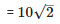Therefore, perimeter of the square is 4 × Side = 4 ×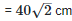103 docs

,

,

,

,

,

,

,

,

,

,

,

,

,

,

,

,

,

,

,

,

,

,

,

,

,

,

,

,

,

,

;